## Saturday, 22 July 2017

### 16-Bit Xorshift Pseudorandom Numbers in Z80 Assembly

Xorshift is a simple, fast pseudorandom number generator developed by George Marsaglia. The generator combines three xorshift operations where a number is exclusive-ored with a shifted copy of itself:

``````/* 16-bit xorshift PRNG */

unsigned xs = 1;

unsigned xorshift( )
{
xs ^= xs << 7;
xs ^= xs >> 9;
xs ^= xs << 8;
return xs;
}
``````

There are 60 shift triplets with the maximum period 216-1. Four triplets pass a series of lightweight randomness tests including randomly plotting various n × n matrices using the high bits, low bits, reversed bits, etc. These are: 6, 7, 13; 7, 9, 8; 7, 9, 13; 9, 7, 13.

7, 9, 8 is the most efficient when implemented in Z80, generating a number in 86 cycles. For comparison the example in C takes approx ~1200 cycles when compiled with HiSoft C v1.3.

``````; 16-bit xorshift pseudorandom number generator
; 20 bytes, 86 cycles (excluding ret)

; returns   hl = pseudorandom number
; corrupts   a

xrnd:
ld hl,1       ; seed must not be 0

ld a,h
rra
ld a,l
rra
xor h
ld h,a
ld a,l
rra
ld a,h
rra
xor l
ld l,a
xor h
ld h,a

ld (xrnd+1),hl

ret
``````## Wednesday, 19 July 2017

### A Fast Z80 Integer Square Root

A project I'm working on needs a fast square root but I couldn't find anything suitable online. After implementing several versions of the bit-by-bit algorithm I discovered the following code is particularly efficient when unrolled:

``````/* Return the square root of numb */

int isqrt( numb )
{
int root = 0, bit = 04000h;
while ( bit != 0 )
{
if ( numb >= root + bit )
{
numb = numb - root - bit;
root = root / 2 + bit;
}
else
root = root / 2;
bit = bit / 4;
}
return root;
}
``````

### First Make It Work

The looping version is small but clunky. It spends almost 400 t-states shifting bits. We'll be able to eliminate most of the shifting by hard-coding the bit positions when the loop is unrolled:

``````; 16-bit integer square root
; 34 bytes, 1005-1101 cycles (average 1053)

; call with de = number to square root
; returns   hl = square root
; corrupts  bc, de

ld bc,08000h
ld h,c
ld l,c
sqrloop:
srl b
rr c
ex de,hl
sbc hl,de
jr c,sqrbit
ex de,hl
jr sqrfi
sqrbit:
ex de,hl
or a
sbc hl,bc
sqrfi:
srl h
rr l
srl b
rr c
jr nc,sqrloop
``````

### Then Make It Work Faster

First the loop is unrolled. The first 4 iterations are optimized to work on 8-bit values and bit positions are hard-coded. The first and last iteration are optimized as a special case, we work with the bitwise complement of the root instead of the root and small jumps are replace with overlapping code. The final code finds the root in an average of 362 t-states:

``````; fast 16-bit integer square root
; 92 bytes, 344-379 cycles (average 362)
; v2 - 3 t-state optimization spotted by Russ McNulty

; call with hl = number to square root
; returns    a = square root
; corrupts  hl, de

ld a,h
ld de,0B0C0h
jr c,sq7
ld a,h
ld d,0F0h
sq7:

; ----------

jr nc,sq6
res 5,d
db 254
sq6:
sub d
sra d

; ----------

set 2,d
jr nc,sq5
res 3,d
db 254
sq5:
sub d
sra d

; ----------

inc d
jr nc,sq4
res 1,d
db 254
sq4:
sub d
sra d
ld h,a

; ----------

jr nc,sq3
ld e,040h
db 210
sq3:
sbc hl,de
sra d
ld a,e
rra

; ----------

or 010h
ld e,a
jr nc,sq2
and 0DFh
db 218
sq2:
sbc hl,de
sra d
rra

; ----------

or 04h
ld e,a
jr nc,sq1
and 0F7h
db 218
sq1:
sbc hl,de
sra d
rra

; ----------

inc a
ld e,a
jr nc,sq0
and 0FDh
sq0:
sra d
rra
cpl
``````

## Saturday, 20 May 2017

### ZX Spectrum BASIC Challenges

Recently I've entered a few of the programming challenges in the BASIC on the ZX Spectrum group on Facebook. They vary in difficultly but it's possible to write a program for most in under 30 minutes. If you're looking for a quick challenge or the opportunity to improve you BASIC, why not take a look.

Here are some examples of the challenges:

### Japanese Pattern

Uwe Geiken asked us to recreate an intricate Japanese pattern. By mirroring, rotating and repeating a 4 line candy cane shape I squeezed this into 156 bytes.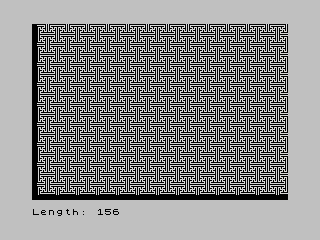### Earth/Venus Orbits

David Saphier challenged us to write the fastest code to display the Earth and Venus orbit pattern in BASIC. Being a bit of a rebel I aimed to write the shortest code and managed to completely botch it before coming up with this working version: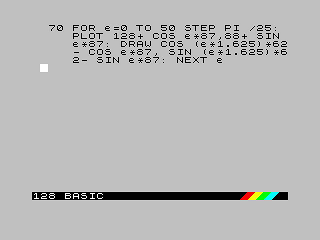### Greenlandic Flag

Matthew Logue issued a challenge to accurately display the flag of Greenland. The flag is simple enough to be reduced to a formula `x²+y² < 54² ⊻ y > 0`: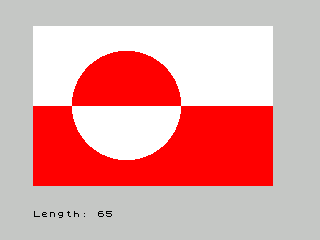### Triangles

Uke Geiken showed a pattern of triangles and asked for the shortest code. The shortest implementation I found uses UDGs:### Grid

Matthew Logue asked us recreate a grid-like pattern with the shortest code. Surprisingly I actually managed to discover the smallest program: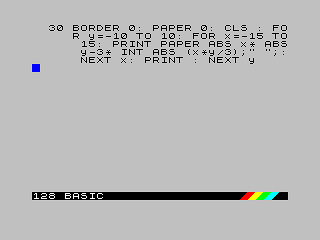### Weaving

‎Uwe Geiken challenged us to recreate a pattern of weaving attributes. I found this one pretty tricky to reduce in size, but finally got it down to 109 bytes: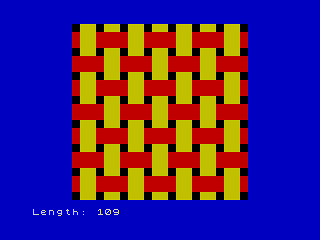### Flag

Matthew Logue asked for the shortest code to recreate a 31×21 attribute flag-like pattern. Uwe Geiken solved this is 67 bytes, easily beating my 74: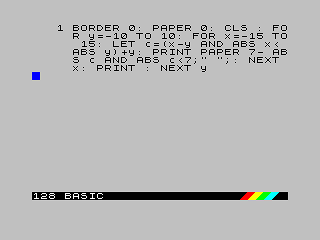### Rudimentary Gear

Matthew Logue challenged us again, this time to draw a rudimentary gear with 10 teeth and a circular radius. Here's what I came up with: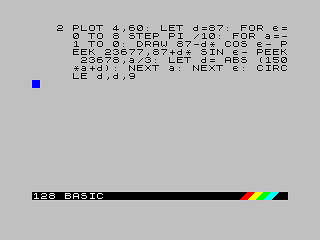## Saturday, 29 April 2017

### ZX Spectrum Scanline Flood Fill

A flood fill is a graphical algorithm to colour an area of screen bounded by pixels of another colour. The scanline technique is a fast, stack-efficient flood fill which can be implemented in 99 bytes of Z80, as demonstrated below:
``````; scanline fill by John Metcalf
; call with d=x-coord, e=y-coord

; set end marker

fill:
ld l,255
push hl

; calculate bit position of pixel

nextrun:
ld a,d
and 7
inc a
ld b,a
ld a,1
bitpos:
rrca
djnz bitpos
ld c,b
ld b,a

; move left until hitting a set pixel or the screen edge

seekleft:
ld a,d
or a
jr z,goright
dec d
rlc b
call scrpos
jr nz,seekleft

; move right until hitting a set pixel or the screen edge,
; setting pixels as we go. Check rows above and below and
; save their coordinates to fill later if necessary

seekright:
rrc b
inc d
jr z,rightedge
goright:
call scrpos
jr z,rightedge
ld (hl),a
inc e
dec e
dec e
inc e
jr seekright

; check to see if there's another row waiting to be filled

rightedge:
pop de
ld a,e
inc a
jr nz,nextrun
ret

; calculate the pixel address and whether or not it's set

scrpos:
ld a,e
and 248
rra
scf
rra
rra
ld l,a
xor e
and 248
xor e
ld h,a
ld a,l
xor d
and 7
xor d
rrca
rrca
rrca
ld l,a
ld a,b
or (hl)
cp (hl)
ret

; check and save the coordinates of an adjacent row

sla c
ld a,e
cp 192
ret nc
call scrpos+1
ret z
inc c
bit 2,c
ret nz
pop hl
push de
jp (hl)
``````

## Sunday, 29 May 2016

### Divide and Conquer Line Algorithm for the ZX Spectrum

While attempting to write a game in 256 bytes I needed a routine to draw lines, but Bresenham's line algorithm weighs in at approx ~120 bytes. The only suitable alternative I'm aware of is recursive divide and conquer: divide a line into two smaller lines and call the draw routine with each in turn:

``````/* Draw a line from (ax,ay) to (bx,by) */

int draw ( ax, ay, bx, by )
{
int midx, midy;
midx = ( ax+bx ) / 2;
midy = ( ay+by ) / 2;
if ( midx != ax && midy != ay )
{
draw( midx, midy, ax, ay );
draw( bx, by, midx, midy );
plot( midx, midy );
}
}
``````

This is significantly smaller thank Bresenham's, 32 byte of Z80. However, there are a couple of compromises: it's slower and the lines aren't perfect because the rounding errors accumulate.

``````; draw lines using recursive divide and conquer
; from de = end1 (d = x-axis, e = y-axis)
; to   hl = end2 (h = x-axis, l = y-axis)

DRAW:
call PLOT

push hl

; calculate hl = centre pixel

ld a,l
rra
ld l,a
ld a,h
rra
ld h,a

; if de (end1) = hl (centre) then we're done

or a
sbc hl,de
jr z,EXIT

ex de,hl
call DRAW    ; de = centre, hl = end1
ex (sp),hl
ex de,hl
call DRAW    ; de = end2, hl = centre

ex de,hl
pop de
ret

EXIT:
pop hl
ret

; ---------------------------

; plot d = x-axis, e = y-axis

PLOT:
push hl
ld a,d
and 7
ld b,a
inc b
ld a,e
rra
scf
rra
or a
rra
ld l,a
xor e
and 248
xor e
ld h,a
ld a,l
xor d
and 7
xor d
rrca
rrca
rrca
ld l,a
ld a,1
PLOTBIT:
rrca
djnz PLOTBIT
or (hl)
ld (hl),a
pop hl
ret
``````

Alternatively the `de(end1) = hl(centre)` test can be replaced with a recursion depth count to create an even slower 28 byte routine:

``````; draw lines using recursive divide and conquer
; from de = end1 (d = x-axis, e = y-axis)
; to   hl = end2 (h = x-axis, l = y-axis)

DRAW:
ld c,8

DRAW2:
dec c
jr z,EXIT

push de

; calculate de = centre pixel

ld a,l
rra
ld e,a
ld a,h
rra
ld d,a

call DRAW2   ; de = centre, hl = end1
ex (sp),hl
call DRAW2   ; de = centre, hl = end2

call PLOT
ex de,hl
pop hl
EXIT:
inc c
ret
``````## Friday, 27 May 2016

### Langton's Ant for the ZX Spectrum

Langton's Ant is an automata which creates a complex pattern by following a couple of simple rules:

• If the ant is on an empty pixel, turn 90° right, set the pixel then move forward
• If the ant is on a set pixel, turn 90° left, reset the pixel then move forward

The ant's path appears chaotic at first before falling into a repetitive “highway” pattern, moving 2 pixels diagonally every 104 cycles.

Here's the code to display Langton's Ant on the ZX Spectrum in 61 bytes. It runs in just over a second so you might want to add a `halt` to slow things down:

``````  org 65472

ld de,128*256+96

ANT:
; halt
ld a,c      ; check direction
and 3
rrca
dec a
jr nc,XMOVE

add a,e     ; adjust y position +/-1
ld e,a
cp 192
ret nc
xor a

XMOVE:
add a,d     ; adjust x position +/-1
ld d,a

; ----------
and 7       ; calculate screen address
ld b,a
inc b
ld a,e
rra
scf
rra
or a
rra
ld l,a
xor e
and 248
xor e
ld h,a
ld a,d
xor l
and 7
xor d
rrca
rrca
rrca
ld l,a
ld a,1
PLOTBIT:
rrca
djnz PLOTBIT
; ----------

ld b,a      ; test pixel
and (hl)

jr nz,LEFT  ; turn left/right
inc c
inc c
LEFT:
dec c

ld a,b      ; flip pixel
xor (hl)
ld (hl),a
jr ANT
``````## Saturday, 3 October 2015

### The Matrix Digital Rain for the ZX Spectrum

A few days ago I coded The Matrix digital rain effect, a fictional representation of the code for the virtual reality of The Matrix. The technique is simple: fill the screen with random characters and scroll down columns of attributes, occasionally switching between black and green.

Here's the final code - 147 bytes of Z80 using the default Sinclair font:

``````        org 08000h

; black border / black attributes

xor a
out (0FEh),a
ld hl,05AFFh
attr:   ld (hl),a
dec hl
bit 2,h
jr z,attr

; fill screen with random characters

ld e,a
fillscr:ld d,040h
fill:   call rndchar
ld a,d
cp 058h
jr nz,fill
inc e
jr nz,fillscr

; digital rain loop

frame:  ld b,06h
halt
column: push bc

; randomize one character

call random
and 018h
jr z,docol
ld d,a
call random
ld e,a
call rndchar

; select a random column

docol:  call random
and 01Fh
ld l,a
ld h,058h

; ~1% chance black -> white

ld a,(hl)
or a
ld bc,0247h
jr z,check

; white -> bright green

white:  cp c
ld c,044h
jr z,movecol

; bright green -> green

cp c
ld c,04h
jr z,movecol

; ~6% chance green -> black

ld bc,0F00h
check:  call random
cp b
jr c,movecol
ld c,(hl)

; move column down

movecol:ld de,020h
ld b,018h
down:   ld a,(hl)
ld (hl),c
ld c,a
djnz down
pop bc
djnz column

; test for keypress

ld bc,07FFEh
in a,(c)
rrca
jr c,frame
ret

; display a random glyph

rndchar:call random
crange: sub 05Fh
jr nc,crange
ld l,a
ld h,0
ld bc,(05C36h)
ld b,8
char:   ld a,(hl)
ld (de),a
inc d
inc hl
djnz char
ret

; get a byte from the ROM

random: push hl
ld hl,(seed)
inc hl
ld a,h
and 01Fh
ld h,a
ld (seed),hl
ld a,(hl)
pop hl
ret

seed:
``````